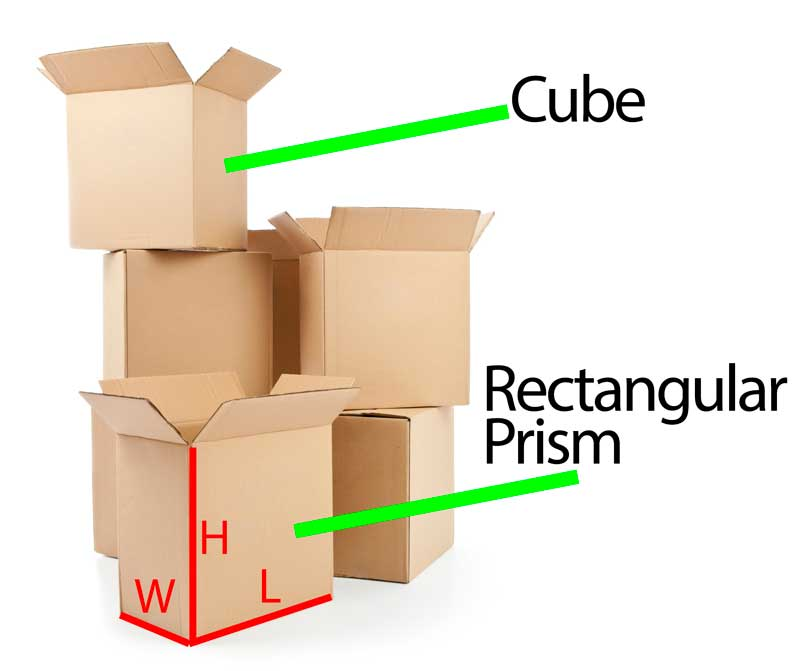# Volume of a Rectangular Prism

A prism with bases that are all rectangles is called a Rectangular Prism. A cube is a special rectangular prism with all its faces congruent. Rectangular prisms are described by its dimensions: length, width, and height.Volume is the number of cubic units it takes to fill a figure.

Watch the video, as a box (rectangular prism) is filled with linking cubes.

How many linking cubes were used to fill the box (rectangular prism)?

How many cubes were used to represent the length of the box?

How many cubes were used to represent the width of the box?

Since you know the total number of the cubes in the box, how could you use this information to help you find the number of cubes it would take to represent the height of the box?

# Volume of a Rectangular Prism

Explore volume of rectangular prisms:

Cubes Interactive
• Click on the “Instructions” drop down menu. Read all of the instruction on how to use the buttons in the applet.
• Explore the volume of the following three rectangular prisms and record your answer to each question in your journal:
• Explore a 5 unit by 4 unit by 3 unit rectangular prism.
How many cubic units are in each layer?
How many layers are needed to fill the rectangular prism?
How many cubic units are needed to fill a rectangular prism that measures 5 unit by 4 unit by 3 unit?
• Explore a 7 unit by 4 unit by 5 unit rectangular prism.
How many cubic units are in each layer?
How many layers are needed to fill the rectangular prism?
How many cubic units are needed to fill a rectangular prism that measures 7 unit by 4 unit by 5 unit?
• Explore a 3 unit by 5 unit by 2 unit rectangular prism.
How many cubic units are in each layer?
How many layers are needed to fill the rectangular prism?
How many cubic units are needed to fill a rectangular prism that measures 3 unit by 5 unit by 2 unit?

The formula for the volume of a rectangular prism is V = l $x$$x$ h.

Respond to the following prompt in your journal:

How is the formula for the volume of a rectangular prism related to its dimensions?